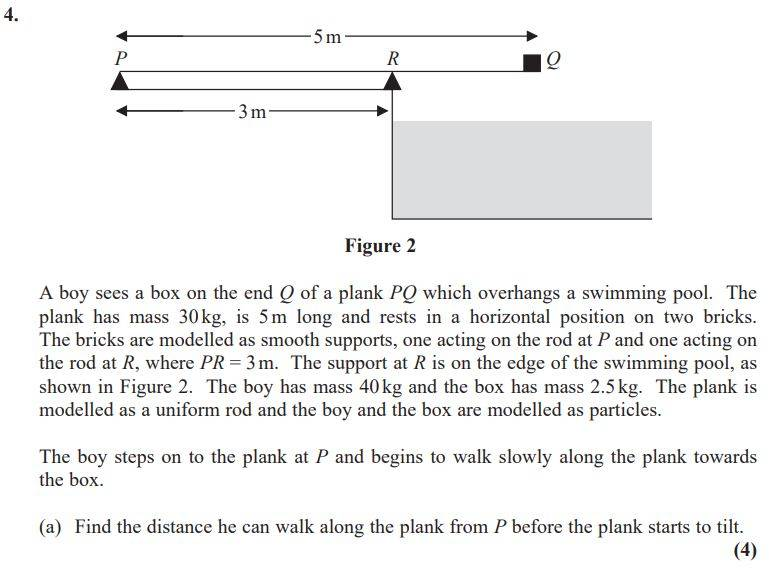# Moments about a point

Homework Statement:
as below
Relevant Equations:
as belowSo I let x be the distance from P.

I then considered moments about R and got this:

1) (40g)(x-3) = 40gx - 120g and this is anticlockwise
2) (30g)(0.5) = 15g and this is anticlockwise
3) (2.5g)(2) = 5g and this is clockwise

And then by equating clockwise and anticlockwise I get this:

40gx - 120g + 15g = 5g

40gx = 110g

x = 110/44
x = 2.75m

But the mark scheme says this is wrong? They've got it as 3.25m. This is what they used as moments about R:

(40g)(x-3) + (2.5g)(2) = (30g)(0.5)

I don't get how they can consider the boy and the box Q to be on the same side??

## Answers and Replies

haruspex
Science Advisor
Homework Helper
Gold Member
2020 Award
1) (40g)(x-3) = 40gx - 120g and this is anticlockwise
Is it?
I don't get how they can consider the boy and the box Q to be on the same side??
When x>3 (40g)(x-3) is positive. Which way will the torque be?

•trew
Is it?

When x>3 (40g)(x-3) is positive. Which way will the torque be?

So when x>3, this will be on the right hand side of the point R making it clockwise, but why do I assume that the boy stands on the right hand side of R?

haruspex
Science Advisor
Homework Helper
Gold Member
2020 Award
why do I assume that the boy stands on the right hand side of R?
You are not making that assumption. When x > 3, the boy is on the right and the torque he exerts about the fulcrum is clockwise; when x < 3 the torque is still "(40g)(x-3) clockwise" because that value is negative, and a negative value for a clockwise torque means it is actually an anticlockwise torque, which it will be.

•trew